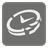Broken Clock
Simple+
English ES JA RUWe have a broken clock. We know how quickly it runs or lags over a specific period of time. At first, the clock is set to the correct time, but after a while it begins to display an incorrect time... But instead of correcting the clock each time, we will use an algorithm to calculate the correct time by accounting for the difference compared to the actual current time. Of course we will have access to the correct time for each day. In addition, you can be certain that the correct starting time and current actual time fall on the same day. For this mission, time is measured in a 24 hour format.

You are given three values. The first is the correct starting time. The second is the current time displayed on the broken clock (which is incorrect). Time is given as strings in the format "hh:mm:ss" (Examples: "01:16:59" and "23:00:13"). The third value is a description of the clock error in the format "+(-)N [second, minute, hour](s) at M [second, minute, hour](s)". For Example "+1 second at 10 seconds" -- the clock is 1 second fast for every 10 seconds of actual time and "-5 minutes at 5 hours" -- the clock lags 5 minutes for every 5 hours of actual time.

You should calculate the real time with the given values. The result should be rounded down to the nearest second (use floor or int).

Let's examine one example -- '00:00:00', '00:00:30', '+2 seconds at 6 seconds'.
0th step: The real and fake time is "00:00:00".
When the real time is "00:00:06", the fake time is "00:00:08".
At real "00:00:18", fake is "00:00:24".
At real "00:00:21", fake is "00:00:28".
At real "00:00:22", fake is "00:00:29.333...".
At real "00:00:22.5", fake is "00:00:30".
So answer is "00:00:22.5" after rounding down "00:00:22"

Input: Three arguments. Correct starting time, current wrong time and broken clock descriptions as strings.

Output: The real time as a string.

Example:
```broken_clock('00:00:00', '00:00:15', '+5 seconds at 10 seconds') ==  '00:00:10'
broken_clock('06:10:00', '06:10:15', '-5 seconds at 10 seconds') ==  '06:10:30'
broken_clock('13:00:00', '14:01:00', '+1 second at 1 minute') ==  '14:00:00'
broken_clock('01:05:05', '04:05:05', '-1 hour at 2 hours') ==  '07:05:05'
broken_clock('00:00:00', '00:00:30', '+2 seconds at 6 seconds') ==  '00:00:22'
```

How it is used: Believe it or not, this mission is teaching you some of the same principles used in GPS tracking. Because the satellites in orbit are moving so fast, we need to account for relativity. So, when we factor in the speed of the satellite, we can figure out the difference from the time on the ground and write a program to compensate.

Precondition:
"wrong_time" is later than "starting_time".